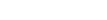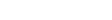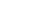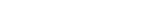【编者按】FAIR研究科学家Tim Rocktäschel简要介绍了einsum表示法的概念，并通过真实例子展示了einsum的表达力。

## 2. Numpy、PyTorch、TensorFlow中的einsum

einsum在numpy中实现为 `np.einsum` ，在PyTorch中实现为 `torch.einsum` ，在TensorFlow中实现为 `tf.einsum` ，均使用一致的签名 `einsum(equation, operands)` ，其中 `equation` 是表示爱因斯坦求和约定的字符串，而 `operands` 则是张量序列（在numpy和TensorFlow中是变长参数列表，而在PyTorch中是列表）。例如，我们的第一个例子，cj = ∑i∑kAikBkj写成 `equation`字符串就是 `ik,kj -> j` 。注意这里 `(i, j, k)` 的命名是任意的，但需要一致。
PyTorch和TensorFlow像numpy支持einsum的好处之一是einsum可以用于神经网络架构的任意计算图，并且可以反向传播。典型的einsum调用格式如下：

2.1 矩阵转置

```import torch
a = torch.arange(6).reshape(2, 3)
torch.einsum('ij->ji', [a])
tensor([[ 0.,  3.],
[ 1.,  4.],
[ 2.,  5.]])```

2.2 求和

```a = torch.arange(6).reshape(2, 3)
torch.einsum('ij->', [a])
tensor(15.)```

2.3 列求和

```a = torch.arange(6).reshape(2, 3)
torch.einsum('ij->j', [a])
tensor([ 3.,  5.,  7.])```

2.4 行求和

```a = torch.arange(6).reshape(2, 3)
torch.einsum('ij->i', [a])
tensor([  3.,  12.])```

2.5 矩阵-向量相乘

```a = torch.arange(6).reshape(2, 3)
b = torch.arange(3)
torch.einsum('ik,k->i', [a, b])
tensor([  5.,  14.])```

2.6 矩阵-矩阵相乘

```a = torch.arange(6).reshape(2, 3)
b = torch.arange(15).reshape(3, 5)
torch.einsum('ik,kj->ij', [a, b])
tensor([[  25.,   28.,   31.,   34.,   37.],
[  70.,   82.,   94.,  106.,  118.]])```

2.7 点积

```a = torch.arange(3)
b = torch.arange(3,6)  # [3, 4, 5]
torch.einsum('i,i->', [a, b])
tensor(14.)```

```a = torch.arange(6).reshape(2, 3)
b = torch.arange(6,12).reshape(2, 3)
torch.einsum('ij,ij->', [a, b])
tensor(145.)```

2.8 哈达玛积

```a = torch.arange(6).reshape(2, 3)
b = torch.arange(6,12).reshape(2, 3)
torch.einsum('ij,ij->ij', [a, b])
tensor([[  0.,   7.,  16.],
[ 27.,  40.,  55.]])```

2.9 外积

```a = torch.arange(3)
b = torch.arange(3,7)
torch.einsum('i,j->ij', [a, b])
tensor([[  0.,   0.,   0.,   0.],
[  3.,   4.,   5.,   6.],
[  6.,   8.,  10.,  12.]])```

2.10 batch矩阵相乘

```a = torch.randn(3,2,5)
b = torch.randn(3,5,3)
torch.einsum('ijk,ikl->ijl', [a, b])
tensor([[[ 1.0886,  0.0214,  1.0690],
[ 2.0626,  3.2655, -0.1465]],
[[-6.9294,  0.7499,  1.2976],
[ 4.2226, -4.5774, -4.8947]],
[[-2.4289, -0.7804,  5.1385],
[ 0.8003,  2.9425,  1.7338]]])```

2.11 张量缩约
batch矩阵相乘是张量缩约的一个特例。比方说，我们有两个张量，一个n阶张量，一个m阶张量。举例来说，我们取n = 4，m = 5，并假定且。我们可以将这两个张量在这两个维度上相乘（A张量的第2、3维度，B张量的3、5维度），最终得到一个新张量，如下所示：

```a = torch.randn(2,3,5,7)
b = torch.randn(11,13,3,17,5)
torch.einsum('pqrs,tuqvr->pstuv', [a, b]).shape
torch.Size([2, 7, 11, 13, 17])```

2.12 双线性变换

```a = torch.randn(2,3)
b = torch.randn(5,3,7)
c = torch.randn(2,7)
torch.einsum('ik,jkl,il->ij', [a, b, c])
tensor([[ 3.8471,  4.7059, -3.0674, -3.2075, -5.2435],
[-3.5961, -5.2622, -4.1195,  5.5899,  0.4632]])```

## 3. 案例

3.1 TreeQN

```import torch.nn.functional as F
return tensors if num == 1 else tensors
# 参数
# -- [激活数 x 隐藏层维度]
# -- [激活数 x 隐藏层维度 x 隐藏层维度]
W = random_tensors([5, 3, 3], requires_grad=True)
def transition(zl):
# -- [batch大小 x 激活数 x 隐藏层维度]
return zl.unsqueeze(1) + F.tanh(torch.einsum("bk,aki->bai", [zl, W]) + b)
# 随机取样仿造输入
# -- [batch大小 x 隐藏层维度]
zl = random_tensors([2, 3])
transition(zl)```

3.2 注意力

```# 参数
# -- [隐藏层维度]
bM, br, w = random_tensors(, num=3, requires_grad=True)
# -- [隐藏层维度 x 隐藏层维度]
WY, Wh, Wr, Wt = random_tensors([7, 7], num=4, requires_grad=True)
# 注意力机制的单次应用
def attention(Y, ht, rt1):
# -- [batch大小 x 隐藏层维度]
tmp = torch.einsum("ik,kl->il", [ht, Wh]) + torch.einsum("ik,kl->il", [rt1, Wr])
Mt = F.tanh(torch.einsum("ijk,kl->ijl", [Y, WY]) + tmp.unsqueeze(1).expand_as(Y) + bM)
# -- [batch大小 x 序列长度]
at = F.softmax(torch.einsum("ijk,k->ij", [Mt, w]))
# -- [batch大小 x 隐藏层维度]
rt = torch.einsum("ijk,ij->ik", [Y, at]) + F.tanh(torch.einsum("ij,jk->ik", [rt1, Wt]) + br)
# -- [batch大小 x 隐藏层维度], [batch大小 x 序列维度]
return rt, at
# 取样仿造输入
# -- [batch大小 x 序列长度 x 隐藏层维度]
Y = random_tensors([3, 5, 7])
# -- [batch大小 x 隐藏层维度]
ht, rt1 = random_tensors([3, 7], num=2)
rt, at = attention(Y, ht, rt1)```

## 4. 总结

einsum是 一个函数走天下 ，是处理各种张量操作的瑞士军刀。话虽如此，“einsum满足你一切需要”显然夸大其词了。从上面的真实用例可以看到，我们仍然需要在einsum之外应用非线性和构造额外维度（ `unsqueeze` ）。类似地，分割、连接、索引张量仍然需要应用其他库函数。